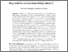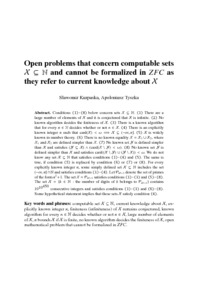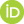# Open problems that concern computable sets X \subseteq N and cannot be formalized in ZFC as they refer to current knowledge about X

Kurpaska, Sławomir and Tyszka, Apoloniusz (2020) Open problems that concern computable sets X \subseteq N and cannot be formalized in ZFC as they refer to current knowledge about X. [Preprint]There is a more recent version of this item available.Preview
Text
open_problems.pdf

## Abstract

Conditions (1)-(8) below concern sets X \subseteq N. (1) There are a large number of elements of X and it is conjectured that X is infinite. (2) No known algorithm decides the finiteness of X. (3) There is a known algorithm that for every n \in N decides whether or not n \in X. (4) There is an explicitly known integer n such that card(X)< \omega ==> X \subseteq (-\infty,n]. (5) X is widely known in number theory. (6) There is no known equality X=X_1 \cup X_2, where X_1 and X_2 are defined simpler than X. (7) No known set Y is defined simpler than X and satisfies (Y \subseteq X) \wedge (card(X\Y)< \omega). (8) No known set Y is defined simpler than X and satisfies card((X\Y) \cup (Y\X))<\omega. We do not know any set X \subseteq N that satisfies conditions (1)-(4) and (5). The same is true, if condition (5) is replaced by condition (6) or (7) or (8). For every explicitly known integer n, some simply defined set X \subseteq N includes the set (-\infty,n] \cap N and satisfies conditions (1)-(4). Let P_{n^2+1} denote the set of primes of the form n^2+1. The set X=P_{n^2+1} satisfies conditions (1)-(3) and (5)-(8). The set X={k \in N: the number of digits of k belongs to P_{n^2+1}} contains 10^{10^{450}} consecutive integers and satisfies conditions (1)-(3) and (6)-(8). Some hypothetical statement implies that these sets X satisfy condition (4).

 Export/Citation: EndNote | BibTeX | Dublin Core | ASCII/Text Citation (Chicago) | HTML Citation | OpenURL
 Social Networking:

Item Type: Preprint
Creators:
CreatorsEmailORCIDTyszka, Apoloniuszrttyszka@cyf-kr.edu.pl0000-0002-2770-5495
Keywords: computable set X \subseteq N, current knowledge about X, explicitly known integer n, finiteness (infiniteness) of X remains conjectured, known algorithm for every n \in N decides whether or not n \in X, large number of elements of X, n bounds X if X is finite, no known algorithm decides the finiteness of X, open mathematical problem that cannot be formalized in ZFC
Subjects: Specific Sciences > Mathematics > Foundations
Specific Sciences > Mathematics > Logic
Depositing User: Apoloniusz Tyszka
Date Deposited: 21 Apr 2020 15:31
Item ID: 17098
Subjects: Specific Sciences > Mathematics > Foundations
Specific Sciences > Mathematics > Logic
Date: 21 April 2020
URI: http://philsci-archive.pitt.edu/id/eprint/17098

### Monthly Views for the past 3 yearsView Item